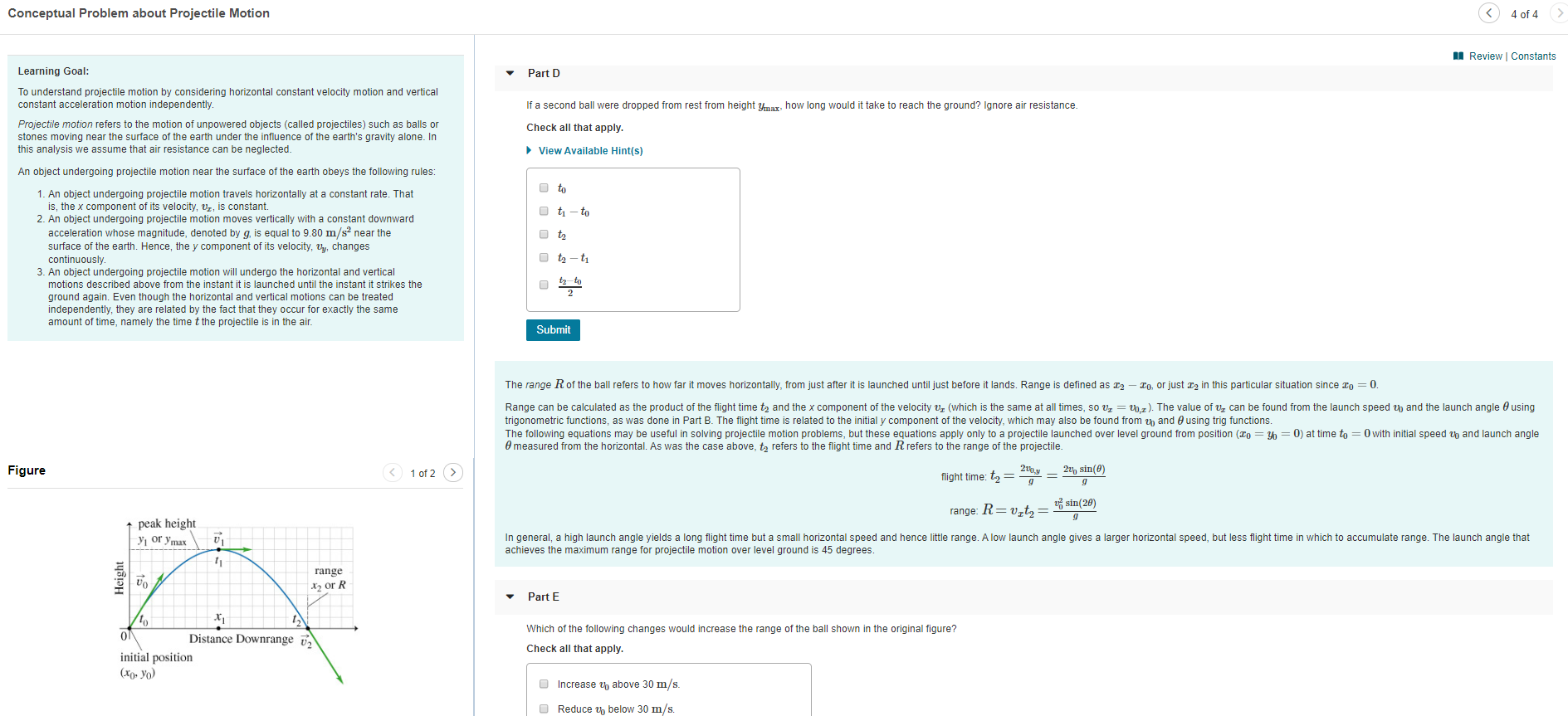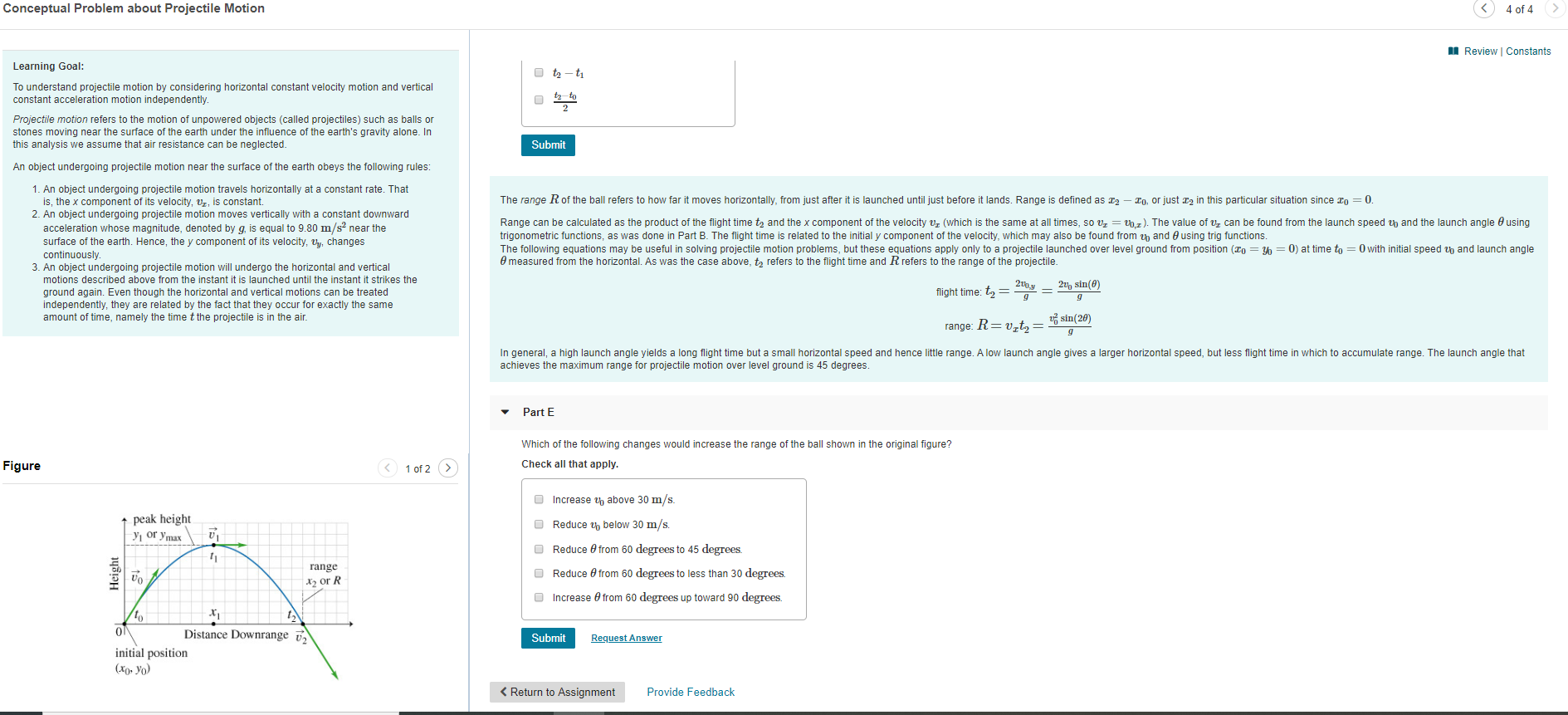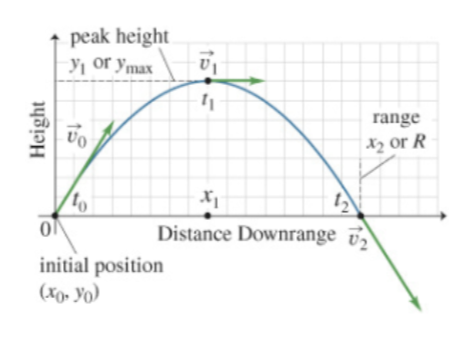# Conceptual Problem about Projectile Motion4 of 4Review | ConstantsLearning Goal:Part DTo understand projectile motion by considering horizontal constant velocity motion and verticalconstant acceleration motion independentlyIf a second ball were dropped from rest from height yma how long would it take to reach the ground? Ignore air resistance.Projectile motion refers to the motion of unpowered objects (callled projectiles) such as balls orstones moving near the surface of the earth under the influence of the earth's gravity alone. Inthis analysis we assume that air resistance can be neglected.Check all that applyView Available Hint(s)An object undergoing projectile motion near the surface of the earth obeys the following rules:to1. An object undergoing projectile motion travels horizontally at a constant rate. Thatis, the x component of its velocity, , is constanttito2. An object undergoing projectile motion moves vertically with a constant downwardacceleration whose magnitude, denoted by g, is equal to 9.80 m/s2 nearsurfacecontinuously3. An object undergoing projectile motion will undergo the horizontal and verticaltthe earth. Hence, the y component of its velocity, uy, changesoft2-tttomotions described above from the instant it is launched until the instant it strikes theground again. Even though the horizontal and vertical motions can be treatedindependently, they are related by the fact that they occur for exactly the sameamount of time, namely the time t the projectile is in the airSubmitthe ball refers to how far it moves horizontally, from just after it is launched until just before it lands. Range is defined asO, or just 2 in this particular situation since o= 0.The rangeRange can be calculated as the product of the flight time ta and the x component of the velocity vr (which is the same at all times, so vr = upz). The value of v, can be found from the launch speed up and the launch angle 0 usingtrigonometric functions, as was done in Part B. The flight time is related to the in itial y component of the velocity, which may also be found from th and e using trig functions.The following equations may be useful in solving projectile motion problems, but these equations apply only to a projectile launched over level ground from position (xo = = 0) at time to 0with initial speed u and launch angleemeasured from the horizontal. As was the case above, t refers to the flight time and R refers to the range of the projectile.2u sin(0)Figure>1 of 2flight time: t=sin(20)range: R t,=t peak heightУт or ymaxIn general, a high launch angle yields a long flight time but a small horizontal speed and hence little range. A low launch angle gives a larger horizontal speed, but less flight time in which to accumulate range. The launch angle thatachieves the maximum range for projectile motion over level ground is 45 degreesrangeX or RPart EWhich of the following changes would increase the range of the ball shown in the original figure?0Distance DownrangeCheck all that apply.initial position(Хо- Уо)Increase u above 30 m/sReduce u below 30 m/s.Height Conceptual Problem about Projectile Motion4 of 4Review | ConstantsLearning Goal:t-tTo understand projectile motion by considering horizontal constant velocity motion and verticaltz toconstant acceleration motion independently.Projectile motion refers to the motion of unpowered objects (called projectiles) such as balls orstones moving near the surface of the earth under the influence of the earth's gravity alone. Inthis analysis we assume that air resistance can be neglectedSubmitAn object undergoing projectile motion near the surface of the earth obeys the following rules:1. An object undergoing projectile motion travels horizontally at a constant rate. Thatis, the x component of its velocity, , is constant2. An object undergoing projectile motion moves vertically with a constant downwardacceleration whose magnitude, denoted by g, is equal to 9.80 m/s2 near thesurface of the earth. Hence, the y component of its velocity, u changescontinuouslyThe range R of the ball refers to how far it moves horizontally, from just after it is launched until just before it lands. Range is defined as -0, or just x2 in this particular situation since ro= 0.Range can be calculated as the product of the flight time ta and the x component of the velocity vr (which is the same at all times, so vr = upz). The value of v, can be found from the launch speed up and the launch angle 0 usingtrigonometric functions, as was done in Part B. The flight time is related to the in itial y component of the velocity, which may also be found from th and using trig functions.The following equations may be useful in solving projectile motion problems, but these equations apply only to a projectile launched over level ground from position (o = Yo = 0) at time to = 0with initial speed u and launch angle0measured from the horizontal. As was the case above, ta refers to the flight time and R refers to the range of the projectile3. An object undergoing projectile motion will undergo the horizontal and verticalmotions described above from the instant it is launched until the instant it strikes the2uo sin(0)2t0yflight time: tground again. Even though the horizontal and vertical motions can be treatedindependently, they are related by the fact that they occur for exactly the sameamount of time, namely the time t the projectile is in the air.sin(20)range: R vtIn general, a high launch angle yields a long flight time but a small horizontal speed and hence little range. A low launch angle gives a larger horizontal speed, but less flight time in which to accumulate range. The launch angle thatachieves the maximum range for projectile motion over level ground is 45 degreesPart EWhich of the following changes would increase the range of the ball shown in the original figure?Check all that apply.Figure1 of 2Increase uh above 30 m/s.peak heightReduce u below 30 m/s.у or ymaxReduce 0 from 60 degrees to 45 degrees.rangeReduce 0 from 60 degrees to less than 30 degreesX2 or RIncrease 0 from 60 degrees up toward 90 degrees.ЛoONDistance DownrangeSubmitRequest Answerinitial position(x- Yo

Question
10 views

How do you solve these? Can you show steps?help_outlineImage TranscriptioncloseConceptual Problem about Projectile Motion 4 of 4 Review | Constants Learning Goal: Part D To understand projectile motion by considering horizontal constant velocity motion and vertical constant acceleration motion independently If a second ball were dropped from rest from height yma how long would it take to reach the ground? Ignore air resistance. Projectile motion refers to the motion of unpowered objects (callled projectiles) such as balls or stones moving near the surface of the earth under the influence of the earth's gravity alone. In this analysis we assume that air resistance can be neglected. Check all that apply View Available Hint(s) An object undergoing projectile motion near the surface of the earth obeys the following rules: to 1. An object undergoing projectile motion travels horizontally at a constant rate. That is, the x component of its velocity, , is constant ti to 2. An object undergoing projectile motion moves vertically with a constant downward acceleration whose magnitude, denoted by g, is equal to 9.80 m/s2 near surface continuously 3. An object undergoing projectile motion will undergo the horizontal and vertical t the earth. Hence, the y component of its velocity, uy, changes of t2-t tto motions described above from the instant it is launched until the instant it strikes the ground again. Even though the horizontal and vertical motions can be treated independently, they are related by the fact that they occur for exactly the same amount of time, namely the time t the projectile is in the air Submit the ball refers to how far it moves horizontally, from just after it is launched until just before it lands. Range is defined as O, or just 2 in this particular situation since o= 0. The range Range can be calculated as the product of the flight time ta and the x component of the velocity vr (which is the same at all times, so vr = upz). The value of v, can be found from the launch speed up and the launch angle 0 using trigonometric functions, as was done in Part B. The flight time is related to the in itial y component of the velocity, which may also be found from th and e using trig functions. The following equations may be useful in solving projectile motion problems, but these equations apply only to a projectile launched over level ground from position (xo = = 0) at time to 0with initial speed u and launch angle emeasured from the horizontal. As was the case above, t refers to the flight time and R refers to the range of the projectile. 2u sin(0) Figure > 1 of 2 flight time: t = sin(20) range: R t,= t peak height Ут or ymax In general, a high launch angle yields a long flight time but a small horizontal speed and hence little range. A low launch angle gives a larger horizontal speed, but less flight time in which to accumulate range. The launch angle that achieves the maximum range for projectile motion over level ground is 45 degrees range X or R Part E Which of the following changes would increase the range of the ball shown in the original figure? 0 Distance Downrange Check all that apply. initial position (Хо- Уо) Increase u above 30 m/s Reduce u below 30 m/s. Height fullscreenhelp_outlineImage TranscriptioncloseConceptual Problem about Projectile Motion 4 of 4 Review | Constants Learning Goal: t-t To understand projectile motion by considering horizontal constant velocity motion and vertical tz to constant acceleration motion independently. Projectile motion refers to the motion of unpowered objects (called projectiles) such as balls or stones moving near the surface of the earth under the influence of the earth's gravity alone. In this analysis we assume that air resistance can be neglected Submit An object undergoing projectile motion near the surface of the earth obeys the following rules: 1. An object undergoing projectile motion travels horizontally at a constant rate. That is, the x component of its velocity, , is constant 2. An object undergoing projectile motion moves vertically with a constant downward acceleration whose magnitude, denoted by g, is equal to 9.80 m/s2 near the surface of the earth. Hence, the y component of its velocity, u changes continuously The range R of the ball refers to how far it moves horizontally, from just after it is launched until just before it lands. Range is defined as -0, or just x2 in this particular situation since ro= 0. Range can be calculated as the product of the flight time ta and the x component of the velocity vr (which is the same at all times, so vr = upz). The value of v, can be found from the launch speed up and the launch angle 0 using trigonometric functions, as was done in Part B. The flight time is related to the in itial y component of the velocity, which may also be found from th and using trig functions. The following equations may be useful in solving projectile motion problems, but these equations apply only to a projectile launched over level ground from position (o = Yo = 0) at time to = 0with initial speed u and launch angle 0measured from the horizontal. As was the case above, ta refers to the flight time and R refers to the range of the projectile 3. An object undergoing projectile motion will undergo the horizontal and vertical motions described above from the instant it is launched until the instant it strikes the 2uo sin(0) 2t0y flight time: t ground again. Even though the horizontal and vertical motions can be treated independently, they are related by the fact that they occur for exactly the same amount of time, namely the time t the projectile is in the air. sin(20) range: R vt In general, a high launch angle yields a long flight time but a small horizontal speed and hence little range. A low launch angle gives a larger horizontal speed, but less flight time in which to accumulate range. The launch angle that achieves the maximum range for projectile motion over level ground is 45 degrees Part E Which of the following changes would increase the range of the ball shown in the original figure? Check all that apply. Figure 1 of 2 Increase uh above 30 m/s. peak height Reduce u below 30 m/s. у or ymax Reduce 0 from 60 degrees to 45 degrees. range Reduce 0 from 60 degrees to less than 30 degrees X2 or R Increase 0 from 60 degrees up toward 90 degrees. Лo ON Distance Downrange Submit Request Answer initial position (x- Yo
check_circle

Step 1

Given,help_outlineImage Transcriptionclosepeak height or ymax range X2or R to Distance Downrange initial position (X Yo Height 1B fullscreen
Step 2

Part (D):

Let the time taken to reach maximum height is ta and time taken to reach the ground from maximum height. So,

Step 3

Now, Time of f...

### Want to see the full answer?

See Solution

#### Want to see this answer and more?

Solutions are written by subject experts who are available 24/7. Questions are typically answered within 1 hour.*

See Solution
*Response times may vary by subject and question.
Tagged in

### Other